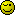# Contact form

Hi,
I have a contact form to submit some words and number, through a script addicarte.php, than index.php
but the index.php have never received the words and number from contenu.php,
the Navigator indicate: http://www.12345.eu/index.php?panier=1
the display is contenu.php page, not the panier.php
I wish someone can help me.

contenu.php

`````` <div id="container">
<input type="hidden" name="ref" value="<?php echo htmlspecialchars(\$r['ref']); ?>"/>
<input type="hidden" name="designation" value="<?php echo htmlspecialchars(\$r['designation']); ?>"/>
<input type="hidden" name="price" value="<?php echo htmlspecialchars(\$r['price']); ?>"/>
<input type="text" name="quantity" size="4"/>
<input type="submit" name="submit" value="confirmer"/>
</form>
</div>
``````

``````<?php
session_start();
if(isset(\$_SESSION['panier'])){
\$panier=\$_SESSION['panier'];
}
else{
\$panier=array();
}
\$index=count(\$panier);
\$panier[\$index]['ref']=\$_POST['ref'];
\$panier[\$index]['price']=\$_POST['price'];
\$panier[\$index]['designation']=\$_POST['designation'];
\$panier[\$index]['quantity']=\$_POST['quantity'];
\$_SESSION['panier']=\$panier;
?>
``````

index.php

``````<div id="prod">
<?php
if(isset(\$_get['panier']))
require_once('panier.php');
else
require_once('contenu.php');
?>
</div>
``````

The script “parts” work as intended posting results to an array and saving to session.

``````Array
(
 => Array
(
[ref] =>
[price] =>
[designation] =>
[quantity] => 4
)

)
``````

What you didn’t show is what array \$r holds, which would populate those hidden fields and give you those “missing words”. Looks more like a cart script than a contact form…

You should edit action in form tag.

Hi, I have a shopping cart, it has problem when I input the quantity, my addcart.php script cannot retain the quantity and the name’s product from DB.
so the display has neither quantity nor product’s name, some one can help me ?

``````<?php
session_start();
if(isset(\$_SESSION['panier'])){
\$panier=\$_SESSION['panier'];
}
else{
\$panier=array();

}
\$index=count(\$panier);
\$panier[\$index]['name']=\$_POST['name'];
\$panier[\$index]['price']=\$_POST['price'];
\$panier[\$index]['quantity']=\$_POST['quantity'];
\$_SESSION['panier']=\$panier;
?>
``````

panier.php

``````<?php
\$panier=\$_SESSION['panier'];

?>

<table border="1">
<tr>
<th>PRODUCT</th><th>PRICE</th><th>QUANTITY</th>
</tr>
<?php
\$total=0;

for(\$i=0;\$i<count(\$panier);\$i++) {
\$total=\$total+\$panier[\$i]['price']*\$panier[\$i]['quantity'];
?>
<tr>
<td><?php echo(\$panier[\$i] ['ref'])?></td>
<td><?php echo(\$panier[\$i] ['price'])?></td>
<td><?php echo(\$panier[\$i] ['quantity'])?></td>
<td><a href="canPanier.php?index=<?=\$i?>">Cancel</a></td>
</tr>

<?php  } ?>

<tr>
<td colspan="3">Total: </td>

<td>
<?php echo(\$total)?>
</td>

</tr>
</table>
``````

product.php

``````  <tr><div id="container">
<td width="76"><span class="col1" align="left"><?php echo htmlspecialchars(\$r['name'])?></td>
<td width="153"><?php echo htmlspecialchars(\$r['price']); ?></td>

<input type="hidden" name="name" value="<?php echo (\$r['name']); ?>"/>
<input type="hidden" name="price" value="<?php echo (\$r['price']); ?>"/>
<input type="text" name="quantity" size="4"/>
<input type="submit" name="submit" value="confirm"/>
</form>
</td>

</div>
</tr>
<?php endwhile; ?>
``````

Do you have session_start(); on every PHP scripts you use? It doesn’t look like you have one in panier.php nor product.php.

Also, try to use the ‘code’ tag in the text editor so that the code you post gets formatted and gets easier to read that wayCan you provide the query loop that wraps around the form?

Or, a copy of view source of the form section of the product page?